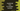# JavaScript program to find if a number is prime or not## What is a prime number :

A number is called prime if that number is divisible by 1 and the number itself. For example, 2, 3, 5, 7 etc. are prime numbers. In this post, I will show you how to check if a number is prime or not in JavaScript with examples.

## Method 1: Using a for loop :

This is the simplest way to check for a prime number. We will write one loop that will iterate from 2 to number/2. For each iteration, we will check if the current loop iteration number can divide the given number or not. If it can divide, it is not a prime. If no number can divide it, it is a prime number.

``````function isPrime(num) {
if (num <= 1) return false;
if (num == 2) return true;

for (let i = 2; i <= num / 2; i++) {
if (num % i == 0) {
return false;
}
}
return true;
}

console.log(isPrime(2));
console.log(isPrime(53));``````

### Explanation :

Here,

• isPrime is used to check if a number is prime or not. It takes one number num as its parameter and returns one boolean value based on num is prime or not.
• If the value of num is equal to or less than 1 return false. If it is 2, return true.
• The for loop checks from 2 to num/2 and if any number can divide num, return false.
• Return true if the loop ends

### Finding all prime numbers from 1 to 100 :

Let’s use the above method to find out all prime numbers from 1 to 100 :

``````function isPrime(num) {
if (num <= 1) return false;
if (num == 2) return true;

for (let i = 2; i <= num / 2; i++) {
if (num % i == 0) {
return false;
}
}
return true;
}

let result = [];

for (let j = 1; j <= 100; j++) {
if (isPrime(j)) {
result.push(j);
}
}

console.log(result);``````

Here, we are calling isPrime method to check if a number is prime or not. The for loop runs from j = 1 to j = 100 and adds all prime numbers to the array result.

It will print the below output :

``````[
2,  3,  5,  7, 11, 13, 17, 19,
23, 29, 31, 37, 41, 43, 47, 53,
59, 61, 67, 71, 73, 79, 83, 89,
97
]``````